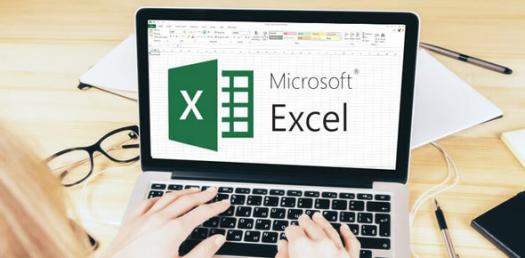# MS. Frazer's Technology Excel Quiz

6 Questions | Total Attempts: 735SettingsThis is a quiz covering the basics of using Microsoft Excel.

• 1.
Why is Excel a useful program?
• A.

Who knows? Ms. Frazer just told me to use it. I don't ask questions!

• B.

It helps keep data organized.

• C.

It can perform calculations.

• D.

It can easily create graphs and charts from data.

• E.

B, C and D

• 2.
True or False: Excel can calculate averages and sums.
• A.

True.

• B.

False.

• 3.
How many different types of charts can you make in Excel?
• A.

One - Column Chart.

• B.

Two - Column Chart and Bar Graph.

• C.

Three - Column Chart, Bar Graph, Pie Chart.

• D.

Many!!

• 4.
If you want to add a number of cells together, what is the formula you use?
• A.

=total(cells)

• B.

=sum(cells)

• C.

• D.

=halloween(cells)

• 5.
How do you change the title of a chart?
• A.

What?! That can't be done!!

• B.

Concentrate really hard, and it will show up.

• C.

In the chart-making wizard.

• 6.
When might you use Excel in the future?
• A.

When organizing data in science.

• B.

When making graphs in math.

• C.

When trying to decide if you're late for carpool.

• D.

A and B.

Related TopicsBack to top